## Introduction

Metamaterials, that is, artificial materials that possess unconventional material parameters, have been employed to achieve unprecedented functionality in the control of electromagnetic and acoustic waves, such as negative refraction1,2,3 and superlensing4,5. One prominent class of metamaterials is anisotropic metamaterials6, the material parameters of which are not scalars but tensors, with their principle components taking different values. This property causes the dispersion relations to display elliptic or hyperbolic shapes7. Such anisotropic metamaterials exhibit distinctive properties, including negative refraction8,9, super-resolution in the far-field through image magnification10 and enhanced spontaneous emission11. When one principle component in the material parameter tensor changes sign, a topological transition occurs12,13. Earlier this year, Luo et al. proposed a method to arbitrarily control electromagnetic flux using a type of anisotropic medium. In this method, only one principle component is near zero and the other components take positive values14. However, a real sample of such a medium is yet to be reported.

The unconventional material parameters of a metamaterial are based on the following two premises: (1) the structure has a subwavelength nature and (2) the metamaterial has local resonances in its building blocks. The subwavelength scale allows the heterogeneous material to be considered as a homogenized effective medium, whereas local resonances lead to exotic values of the effective medium parameters that are rarely or never observed in nature. The existence of resonances poses a considerable challenge to conventional effective medium theories (EMTs), such as the well-known Maxwell-Garnett theory and the Bruggeman theory15. This is because the basic principle of a conventional EMT is to minimize the scattering at the quasi-static limit, while the local resonances usually occur in or even beyond the long-wavelength regime. In the long-wavelength regime, the wavelength in the host medium (λ0) is large compared to the size of the unit cell, but the wavelength in the scatterer (λs) can be very small16. In contrast, both λ0 and λs should be much larger than the size of the unit cell in the quasi-static limit.

Efforts have been made to extend conventional EMTs to higher frequency (or short wavelength) regimes. For example, a coherent potential approximation (CPA) method has been applied to both electromagnetic and elastic waves to enlarge the applicability range of the EMTs17,18. Equivalent results were also obtained by taking full account of the interactions among the scatterers using the multiple-scattering formalism19,20. In addition, a rigorous approach based on the Floquet representation was proposed to homogenize metamaterials with periodic arrays of dielectric inclusions21,22,23. Later, this approach was generalized to incorporate both dielectric and magnetic materials and a first-principles homogenization scheme was developed from dyadic Green's functions and polarizability coefficients. An analytical solution was obtained for periodic systems with isotropic unit cells24,25. Very recently, a method based on reproducing the lowest orders of scattering amplitudes from a finite volume of metamaterials was proposed. This can give accurate predictions of the effective medium parameters over almost the entire Brillouin zone26. These schemes work well for isotropic media in which both the scatterers and the lattice structures are isotropic. For anisotropic media, however, the homogenization scheme is more challenging, as it involves more degrees of freedom than in the case of isotropic media. Many conventional anisotropic EMTs are extensions of the Maxwell-Garnett theory7,27,28,29 and are consequently limited in application to the quasi-static regime. A multiple-scattering-based scheme has been introduced to study the effective medium properties of metamaterials with anisotropic lattices and isotropic scatterers30,31, yielding a scalar bulk modulus and tensorial mass density at finite frequencies in the long-wavelength regime. There exist other schemes that are also applicable to anisotropic scatterers, including the field-averaging32,33, boundary-integration13,34 and parameter-retrieving methods35,36,37,38. The field-averaging and boundary-integration methods require prior knowledge of field distributions, while the parameter-retrieving method requires information about the transmission and reflection coefficients and may give non-unique solutions. More importantly, none of these three methods offer a closed-form analytical solution that can directly predict reliable effective medium parameters from the material and geometric information of the system.

In this work, we consider a rectangular array of elliptic cylinders embedded in air and study its scattering properties. We discover that the special properties of elliptic coordinates and Mathieu functions (solutions to Helmholtz equations in elliptic coordinates) enable us to derive a closed-form analytical solution for the anisotropic effective medium parameters, provided the aspect ratio of the lattice and the eccentricity of the elliptic cylinder satisfy certain conditions. We verify the derived EMT by comparing its predictions with full-wave band-structure simulations and excellent agreements are found at finite frequencies beyond the long-wavelength limit. This new EMT suggests promising opportunities to expand the design of anisotropic metamaterials. We show that a recently theoretically proposed anisotropic near-zero material, which can manipulate electromagnetic flux, can be achieved from the predictions of the derived EMT. The metamaterial is composed of common dielectric materials with simple structures, which makes the fabrication process feasible and would therefore greatly benefit the practical realization of the material.

## Results

### Modeling and the analytical solution

The system considered in our study is a two-dimensional (2D) metamaterial consisting of a periodic rectangular array of elliptic cylinders with permittivity, εs and magnetic permeability, μs, embedded in a background material with permittivity, ε0 and magnetic permeability, μ0. A unit cell of the metamaterial is illustrated in Fig. 1(a). The elliptic cylinder's semi-major and semi-minor axes are as and bs, respectively and its filling ratio, i.e., the ratio of the area of the elliptic cylinder to the area of the unit cell, is f. Given as, bs and f, the length, a and the width, b, of the unit cell are determined by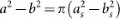and abf = πasbs. For the dispersion microstructure15, in which the scatterers are always dispersed in the matrix, the CPA scheme considers the scattering of a coated cylinder in an effective medium (as shown in Fig. 1(b)). The inner elliptic cylinder represents the scatterer in the metamaterial and the coating layer is the background medium, while the semi-major and semi-minor axes of the outer elliptic cylinder are a0 and b0, respectively. Such a coated elliptic cylinder represents the microstructure of the metamaterial or the average cell16 in the CPA scheme, as the outside environment has been averaged as an effective medium. It is generalized from the circular (or spherical) average cells of isotropic lattices with isotropic scatterers17,39. The cross-sectional area of the average cell should be identical to that of a unit cell, i.e., πa0b0 = ab, so that the filling fraction of the scatterer in the average cell is fixed to that of the metamaterial. The aspect ratio of the average cell should also equal that of the rectangular unit cell, i.e., a0/b0 = a/b, in order to preserve the symmetry properties. With this average cell, the anisotropic property of the metamaterial is maintained and the scattering property in the effective medium is correctly produced. The effective medium parameters (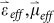) are obtained when the total scattering of the average cell vanishes in the limit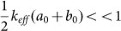. In the Method section, we present detailed steps to derive the EMT of the metamaterial for a transverse-electric (TE)-polarized wave, in which the electric field is parallel to the elliptic cylinders (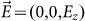). Here, we refer only to the final solutions, which are expressed as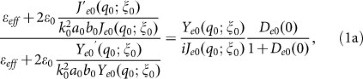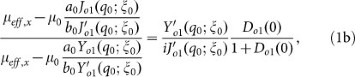and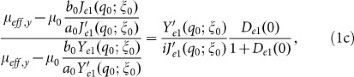where the effective permittivity, εeff, is a scalar related to the monopolar mode and the effective permeability is a tensor whose principle components μeff,x and μeff,y are associated with the y-polarized and x-polarized dipolar modes, respectively. The x- and y-axes are set in the directions of the semi-major and semi-minor axes of the elliptic cylinders, respectively. Note that all of the notations and subscripts appearing in Eq. (1) are defined or introduced in the Method section.

### Verification of the EMT

In Fig. 2(a), we plot the band structure of a metamaterial obtained from a full-wave simulation using black dots. The metamaterial is composed of elliptic cylinders in a rectangular lattice embedded in air and the geometric sizes of the scatterer and the lattice are as = 0.26r, bs = 0.2r, a = 1.16r and b = 1.12r, where r is a normalized length unit. The material parameters are chosen as εs = 12, μs = 1 for the scatterer and ε0 = 1, μ0 = 1 for air. Also plotted in Fig. 2(a) (in red solid curves) are the band structures predicted by the EMT, i.e., Eq. (1). The corresponding effective permittivity and permeability are shown in Figs. 2(b) and 2(c), respectively. They provide us with a clear picture and understanding of the dispersion relations. We label three points on the band structure at the Brillouin zone center as “A”, “B” and “C” (the blue dots in Fig. 2(a)). The eigenfrequencies of these points are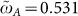,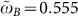and, respectively and the dimensionless frequency,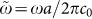, is used (c0 is the wave velocity in air). Comparing Fig. 2(a) with Figs. 2(b) and 2(c), we find that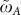,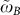and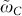correspond exactly to the frequencies at which μeff,y, εeff and μeff,x become zero. Because the dispersion relations of such an anisotropic medium are determined by7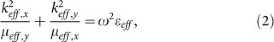it is easy to obtain the dispersion relations in different directions. For example, in the ΓXY) direction, i.e., keff,y = 0 (keff,x = 0), we have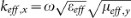(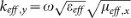). If both εeff and μeff,y are positive (negative) over a frequency range, then there is a positive (negative) band in the ΓX direction. If these two quantities have different signs, then there is a gap in the ΓX direction rather than a pass band. The same rules apply to the dispersion relations along the ΓY direction if we replace μeff,y with μeff,x. With these rules, all the dispersion relations near points “A”, “B” and “C” can be easily interpreted. For example, for frequencies between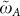and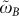, both εeff and μeff,x are negative and μeff,y is positive. Thus, there is a negative band in the ΓY direction, but a gap in the ΓX direction. When the frequency is slightly higher and located between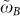and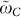, both εeff and μeff,y are positive and μeff,x is negative, explaining the positive band in the ΓX direction and the gap in the ΓY direction. The flat bands near points “A” and “C” in the ΓY and ΓX directions are in fact the longitudinal bands induced by μeff,y and μeff,x equal to zero17, respectively.

Figure 2(a) illustrates the excellent agreements between the numerical simulations and the derived EMT in the center of the Brillouin zone. We also notice that the red curve deviates from the black dots when the Bloch wave vector is far removed from the Γ point. This is reasonable because we used the condition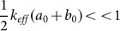in deriving Eq. (1), which limits the range of applicability of the EMT. When the Bloch wave vector is sufficiently large that this condition no longer holds, the EMT is deemed to be inaccurate. Nevertheless, the derived EMT still yields accurate predictions for the effective medium parameters near the Γ point. Note that the red curves coincide with the black dots in the frequency regimes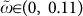and (0.48, 0.61) in the ΓX direction and (0, 0.11) and (0.53, 0.66) in the ΓY direction. We also computed the transmission spectrum of a plane wave normally incident on a 9-layer metamaterial sample embedded in air in these frequency regimes and the results are plotted in Figs. 2(d) (x-direction) and 2(e) (y-direction) using black dots. For comparison, the transmission spectrum of the same sample but with the metamaterial replaced by a slab of effective medium is represented by the red curves, which are calculated from the standard formula of the transmission coefficient of a layered medium40. Good agreements between the numerical simulation and the effective medium prediction are again observed. Since the band structure and transmission coefficients can be used to determine the effective velocity and the impedance of the sample, respectively, Figs. 2(a), 2(d) and 2(e) offer us clear evidence that the EMT is valid.

A systematic study of the applicability of the EMT is presented in the Discussion section. Here we emphasize that, for this case, Eq. (1) is valid even when the dimensionless frequency is as high as 0.66, at which the wavelength in the background medium is 1.52a (or 1.57b), far beyond the quasi-static limit. Figures 2(f)–2(h) illustrate the field distributions of the eigenstates at points “A”, “B” and “C”, which clearly show an x-polarized dipolar mode, a monopolar mode and a y-polarized dipolar mode, respectively. These figures again support the results given by Eq. (1) that μeff,y, εeff and μeff,x are determined by the scattering coefficients of the x-polarized m = 1 mode, the m = 0 mode and the y-polarized m = 1 mode, respectively.

### An anisotropic zero-index metamaterial

As shown in Fig. 2, when the frequency takes values of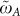,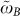and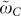, the system can be regarded as an anisotropic zero-index material, because one of the effective material parameters is near zero. Zero-index materials have unprecedented abilities to manipulate electromagnetic waves13,14,41,42,43,44,45. Here, we would like to focus particularly on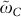, where μeff,x = 0.002→0+, μeff,y = 0.5637μeff,x and εeff = 0.1175. This indicates that the system is an anisotropic zero-index material with only one component of the permeability tensor near zero. Very recently, such a medium was theoretically proposed and found to be capable of cloaking an arbitrarily shaped defect and of exciting evanescent waves near the defect boundaries, which therefore offers a new method of controlling the electromagnetic flux14. Below, we provide simulated results of the wave transmission through such a metamaterial loaded with defects. Figure 3(a) illustrates a schematic picture of the sample, which is a waveguide filled with a metamaterial slab (composed of 12 × 10 previously mentioned unit cells). Three defects labeled “1”, “2” and “3” are distributed within the slab, as shown in Fig. 3(a), with respective sizes of 2a × 2b, 2a × b and 3a × 2b and permeability μ = 1.5, 0.4 and 2.1, respectively. The permittivity of the defects is set to 1. A TE-polarized plane wave with frequency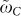is incident from the left.

As a comparison, we plot in Fig. 3(b) the electric field for the same sample shown in Fig. 3(a), but without the metamaterial. Strong scattered waves are excited by the defects, which significantly distort the incident wave fronts. However, the results are significantly altered in the presence of the metamaterial. Figures 3(c), 3(e) and 3(g) show, respectively, the electric field and the magnetic fields in the x- and y-directions. The field patterns at the outlet of the waveguide are almost the same as those of the incident wave, indicating the good cloaking effect of the metamaterial. From Fig. 3(c), we clearly observe an almost uniform field distribution in the y-direction (vertical direction) and an apparent phase change in the x-direction (horizontal direction) in the metamaterial, implying that the metamaterial is highly anisotropic. The wavelength is nearly infinite along the y-direction, but finite along the x-direction. The corresponding field distribution patterns for the same case, but with the metamaterial replaced by the effective medium, are plotted in Figs. 3(d), 3(f) and 3(h). Similar patterns to those shown in Figs. 3(c), 3(e) and 3(g) at the inlet and outlet of the waveguide are seen, suggesting that the EMT indeed describes the physical properties of the metamaterial. From Fig. 3(f), we find that evanescent waves around the defects are induced, which are essential for high transmittance14.

Figure 3 demonstrates the functionality of the anisotropic zero-index metamaterial. Noting that the building blocks of the metamaterial are dielectric elliptic cylinders, which are easily attainable and that there are no complex structures involved, we believe that the fabrication of such a metamaterial is feasible.

## Discussion

We support the validity and application of our anisotropic EMT by illustrating a simulated example, in which a set of values of as/bs, εs, μs and a filling ratio of f = πasbs/ab are chosen and good agreements between the numerical simulations and the EMT predictions are observed. In the following, we conduct a systematic study of the manners in which the material and geometric parameters influence the accuracy of the EMT. In Fig. 4, we plot the frequencies at which zero effective medium parameters are obtained as functions of various parameters. The curves are obtained from Eq. (1) and the dots correspond to the frequencies of the lowest monopolar and dipolar states at the Γ point, which are results of the band structure calculations. In Figs. 4(a)–4(c), we fix the permeability of the scatterers to 1 and change the aspect ratio, permittivity and the filling ratio of the scatterers, respectively. In the lower panel of Fig. 4, we study similar cases to those in the upper panel but with the permittivity of the scatterers fixed at 1. Figure 4 demonstrates that the predictions of our EMT in general coincide with the band structure simulations. When the aspect ratio and filling ratio increase, the predictions deviate from the numerical results. This is reasonable as higher angular momentum terms, i.e., m ≥ 2, contribute to the eigenmodes at low frequencies when the elliptic cylinder becomes flatter or larger. This effect leads to inaccurate predictions, because our derived effective medium scheme does not consider higher angular momentum terms.

In summary, we have derived an anisotropic EMT for a 2D electromagnetic metamaterial. This theory can provide closed-form analytical solutions for anisotropic effective medium parameters and reveal the link between the effective medium parameters and the resonant modes. It is found that the effective permittivity is related to the monopolar mode and the effective permeability tensor is associated with the dipolar modes. The validity of the theory is verified by band structure and transmission spectra calculations and we find that the theory is valid even when the wavelength in the background medium is comparable to the size of the lattice, which is beyond the long-wavelength limit. At the quasi-static limit, our EMT recovers the Maxwell-Garnett formula. We expect that the EMT developed here will facilitate the design of new metamaterials and we show that a recently proposed anisotropic zero-index material can indeed be fabricated from a periodic structure. Additional anisotropic metamaterials with various desired properties may also be devised based on the predictions of our EMT. Although this theory is derived for electromagnetic metamaterials, it can be generalized to its acoustic counterpart because of the mathematical mapping between these two systems in two dimensions.

## Methods

### Solution of the Helmholtz equation in elliptic coordinates

Considering the microstructure shown in Fig. 1(b) for a TE-polarized wave, the electric field in the effective medium can be expressed as46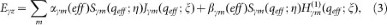and, similarly, the electric field in the background medium of the coating layer is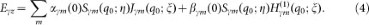Here, η and ξ, where 0 ≤ η < 2π and 0 ≤ ξ < ∞, represent elliptic coordinates that can be transformed into Cartesian coordinates according to x = c cos(η)cosh(ξ)and y = c sin(η)sinh(ξ), where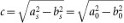represents the focal length of the elliptic coordinate system. In Eqs. (3) and (4), Sm(q; η) denote the angular Mathieu functions of the first kind, while Jm(q; ξ) and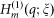are the radial Mathieu functions of the first and third kinds, respectively. The subscript m is an integer denoting the order of the Mathieu functions. The angular and radial Mathieu functions form solutions to the Helmholtz equation in elliptic coordinates, which split into decoupled even (denoted by subscript e) and odd modes (denoted by subscript o) with respect to the x-axis for non-zero m. Here, the general notation γ = e, or o, is used. The variable q0 (qeff) is a dimensionless quantity and is equal to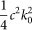(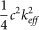), where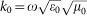(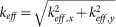) is the wave vector in the background (effective) medium.

### Boundary Conditions

The expansion coefficients in Eqs. (3) and (4), i.e. αγm(σ)and βγm(σ)with σ = 0, or eff, are related through the boundary conditions, which are the continuities of the tangential components of both the electric and magnetic fields on the interface between the background and effective medium. The boundary conditions can be expressed as Ez(eff) = Ez(0) and Hη(eff) = ∂ξEz(0)/μ0 at ξ = ξ0, where ξ0 = cosh−1(a0/c) = sinh−1(b0/c) is the outer boundary of the coated cylinder and Hη(eff) is expressed in the anisotropic effective medium as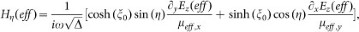with Δ = cosh20)sin2(η) + sinh20)cos2(η). Substituting Eqs. (3) and (4) into the boundary conditions, we obtain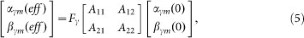where Fγ is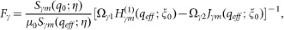and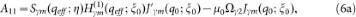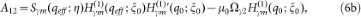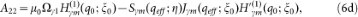where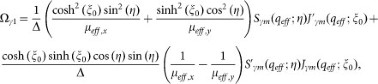and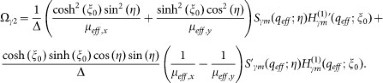### Analytical solution for the effective medium

The effective medium condition requires that the scattering of the coated cylinder vanishes. Since the scattered field of the coated cylinder is represented by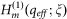, a vanishing scattered wave in the effective medium implies that βγm(eff) = 0. According to Eq. (5), such a condition leads to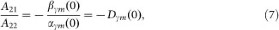where Dγm(0) represent the Mie scattering coefficients of a scatterer of the metamaterial. These coefficients can be obtained by solving the Helmholtz equation and matching the boundary conditions between the scatterer and the background medium. They have the form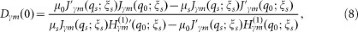in which the subscript “s” means that the quantities take the corresponding values of the scatterer, while ξs indicates the boundary of the scatterer.

When the wavelength in the effective medium is much larger compared to the size of the coated cylinder, i.e.,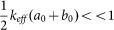, the scattering of the coated cylinder is dominated by monopolar (m = 0) and dipolar (m = 1) terms. Under this condition, we substitute Eqs. (6c) and (6d) into Eq. (7) and approximate the zero- and first-order Mathieu functions associated with the effective medium by Se0(qeff; η) = 1,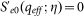, Se1(qeff; η) = cos(η),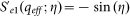, So1(qeff; η) = sin(η),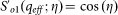, Je0(qeff; ξ0) = 1,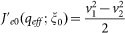,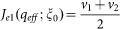,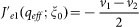,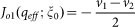and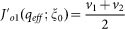, with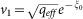and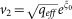. We obtain Eq. (1), i.e.,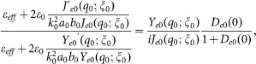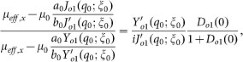and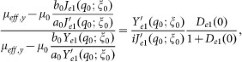where Yγm(q0; ξ0) are the Mathieu Neumann functions. Similar to the results for the isotropic media17, the effective permittivity and permeability are determined by monopolar (m = 0) and dipolar (m = 1) modes, respectively. For the anisotropic case discussed here, however, the effective permeability is no longer a scalar, but a diagonalized tensor. It is interesting to note that the elements of the tensor, μeff,x and μeff,y, correspond exactly to the scattering coefficients of the first-order y-polarized and x-polarized dipolar modes.

### Results in the quasi-static limit

In the quasi-static limit, i.e.,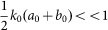and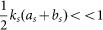, the Mathieu functions in Eq. (1) can be approximated in the same manner as that used to obtain Eq. (1) and the Mathieu Neumann functions. Yγm(qχ; ξχ) and its derivatives,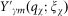, can be approximated as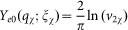,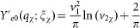,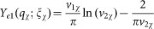,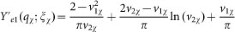,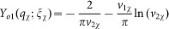and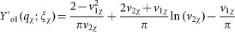, with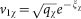and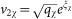, where χ = 0, or s. Notice that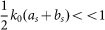is also satisfied in the quasi-static limit. We can treat the corresponding Mathieu Bessel and Neumann functions, as well as their derivatives, Jγm(q0; ξs),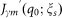, Yγm(q0; ξs) and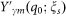, in a similar manner as previously. Eq. (1) can be reduced to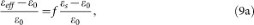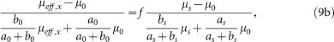and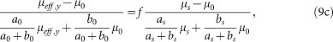where f = asbs/a0b0 is the filling ratio of the elliptic cylinder. It is worth mentioning that Eq. (9) is exactly the Maxwell-Garnett (M-G) version EMT47, in which the effective parameters are functions of the filling ratio and do not depend on the frequency.

### Results in the limit of vanishing eccentricity

In the limit of vanishing eccentricity, i.e., as/bs → 1 (or c → 0), the scatterer becomes an isotropic cylinder and the rectangular lattice correspondingly becomes a square lattice, according to the relation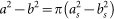. In this limit, the angular and radial Mathieu functions transform into the trigonometric and Bessel functions, respectively46. As a result, Eq. (1) is reduced to Eq. (7) in Ref. 17.

### Numerical simulations

All the numerical simulations presented here are performed using COMSOL Multiphysics, a commercial package based on the finite-element method. Figures 2(a) and 2(f)–2(h) are computed using the eigenfrequency study in the RF module. The Bloch boundary conditions are imposed on the boundaries of the unit cells. The black dots in Figs. 2(d) and 2(e) are calculated using the frequency domain study in the RF module. The same module is used in Figs. 3(b) –3(h). A radiation boundary condition is placed at the waveguide outlet so that there is no reflected wave, while periodic boundary conditions are set on the upper and lower boundaries of the waveguide. The TE-polarized plane wave with frequency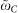is incident from the left.